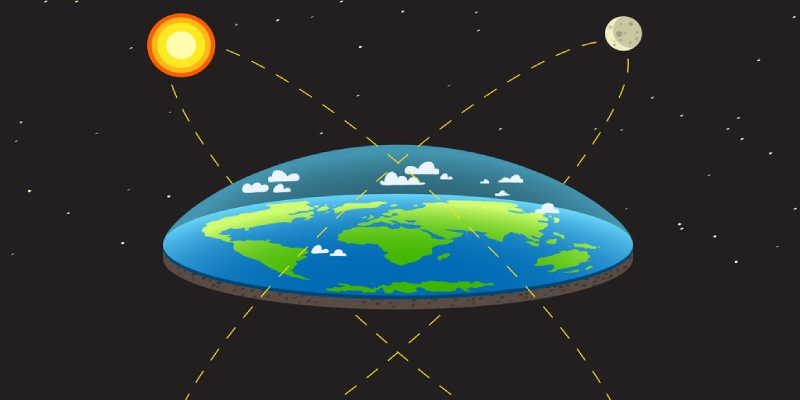### Ultimate Science Trivia Quiz On Gravitation for 9th Grade### Questions & Options

#### 1. The value of acceleration due to gravity......

 75540 Is same on equator and poles 75541 Is least on pole 75542 Is least on equator 75543 Increase from pole to equator

#### 2. Mass per unit volume is called......

 75544 Pressure 75545 Density 75546 Weight 75547 Thrust

#### 3. The force acting on an object perpendicular to the surface is called.....

 75548 Pressure 75549 Density 75550 Thrust 75551 Weight

#### 4. The S.I unit of relative density is.....

 75552 Cm 75553 M 75554 Pa 75555 Unitless

#### 5. The purity of milk is determined by an instrument called.....

 75556 Potentiometer 75557 Lactometer 75558 Hydrometer 75559 Milkometer

#### 6. According to Archimedes's principles, the upward force acting on an object is to be equal to......

 75564 Density of object 75565 Density of water 75566 Weight of fluid displaced 75567 Weight of object inside fluid

#### 7. If a body display more fluid than its weight, it will.....

 75568 Float above the surface of fluid 75569 Float below the surface of fluid 75570 Sink to the bottom of the fluid 75571 Can be anywhere inside the fluid

#### 8. The force exerted by a fluid in the upward direction is called.....

 75572 Pressure 75573 Weight 75574 Buoyant force 75575 None of these

#### 9. The Law of gravitation gives the gravitational force between.....

 75580 The earth and a point mass only 75581 The earth and sun only 75582 Two charged bodies only 75583 Any two bodies having some mass

#### 10. The value of quantity G in the law of gravitation.....

 75584 Depend on mass of earth only 75585 Depend on both mass and radius of earth 75586 Depend on radius of earth only 75587 Is independent of mass and radius of the earth

View All